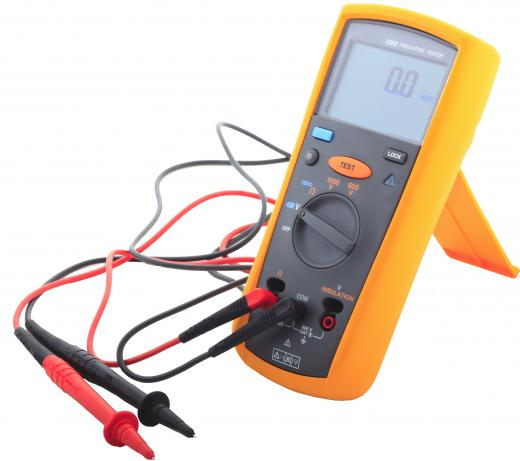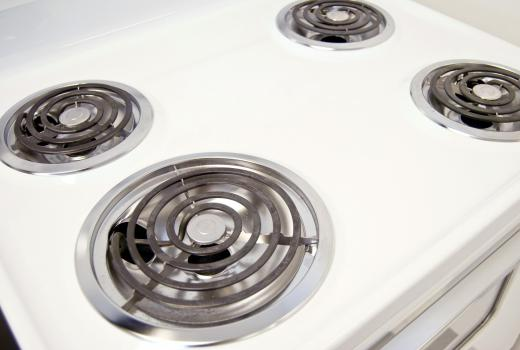# What is the Difference Between an Amp, Volt, and Watt?A multimeter is a tool that can measure amps, volts, and ohms.

Amps, volts, and watts are all ways of measuring different aspects of electricity. An ampere, or amp (A or I, for current), is the amount of current in a circuit, while voltage (V) is the strength of the current as it flows through the circuit, and watts (W) are the total electrical power released by circuit per second. One watt is equal to one volt multiplied by one amp, which can also be expressed as

1 W = 1 V × 1 A

One common analogy used to illustrate these terms is that of a garden hose. Amps represent the rate of water as it flows through the hose, while volts represent the strength of the flow, and the wattage represents the total amount of water that comes out of the hose per second.

#### As Related to Ohms

Another closely related measurement is ohms (Ω), which is a unit of electrical resistance. Going back to the garden hose analogy, if the hose has a larger diameter, more water will be able to flow through. A circuit with a high degree of resistance, expressed in ohms, is able to carry fewer amps than one with lower resistance, regardless of the voltage. If a high voltage encounters high resistance, the amount of possible current in the circuit will be very low — not much water will get through a narrow hose, no matter how high the pressure is. People often express the relationship between current and resistance as Ohm's law:

A - V/Ω

#### In Electrical CircuitsAmps, volts, watts, and ohms measure different aspects of electricity as it travels through a circuit.

Scientists use all of these measurements when working with electric circuits. In their most basic form, electrical circuits consist of a voltage source with a positive and negative terminal, like a battery; a load; and two wires connecting the two. When a current (A) flows out of the negative terminal of a voltage (V) source, it flows towards the positive one through the wires. If it encounters a load in the middle, like a motor or light bulb, it flows through that, releasing power (W), in the form of light or work. The load also provides resistance (Ω), which makes the current flow more slowly.

#### Practical ApplicationsAppliances like stoves that use a large amount of current may have a separate, dedicated circuit.

Understanding how these terms relate to one another can be useful both for performing basic electrical work and determining whether an existing electrical panel can support another appliance. While watts are changeable, voltage is generally fixed, with the US having a 120-volt standard. An appliance that uses a large amount of current, such as an electric stove, may need to be on a separate circuit with a higher voltage. This is because it requires a higher wattage, meaning that it uses up more current per unit of time than other appliances, so it needs more voltage. Without the higher voltage, it wouldn't run, because it would be starved for the current it needed to be able to operate.

Understanding the relationship between various electrical terms can also help consumers evaluate monthly power bills. Electrical consumption is measured in watts, or watt-hours, and power companies measure and bill consumption in kilowatt-hours, which is the equivalent of using 1000 watts of power for one hour. Many households use hundreds of kilowatt-hours each month.

The number of kilowatts billed to each household is connected to volts and amps. Volts remain constant, but amps change based on the demand for current. When people switch on more appliances or run them for longer periods, they increase the demand for current, which in turn increases total watts consumed. By running fewer appliances or switching to more efficient devices that require less current, people can reduce the number of kilowatts consumed and reduce energy bills.anon983458

So if I have a 12v@500mA power supply, can I run 5 units that draw 12v@100mA? A quick response would be much appreciated.

anon302632

Thanks for that. I am a high school dropout, working on Piezoelectricity harvesting. I am one of those guys gets the so called "hard stuff," but lack in the fundamentals of how to get there. This has been very helpful.

anon274994

I do not know the number of Amps of suction power and I want to know if 900 watts is stronger than 12 volts or is it the same?

anon248642

Analogy to garden hose is bad. In physics the smaller the pipe the bigger the pressure which according to your analogy means that bigger resistance causes more bigger current, is that so?

anon213616

complicated theory explained in simple terms. Thank you very much indeed. Douglas S.

anon157854

Explained in very simple way. Very useful.

anon153411

i have a question. i have learned that: watt=volt*amp.

If i have 6v battery and with 4.5ah which means

w=6*4.5 equal to 27, can i operate a 13 watt energy saver? Please answer.

gregh2223

Thanks for the explanation. Here's a question that has puzzled me for a long time about volts and amps: Suppose you get two non-lethal electric shocks. You're in the same place and everything else is the same, including the amount of watts that you were shocked with, except for one factor: in the Shock#1, there were more volts than in Shock#2. That would mean, of course, since the watts are the same, that in Shock#2 there were more amps than in Shock#1. So which shock would be more, umm, shocking, #1 or #2?

anon136138

Good one. Now I can explain it to my kids. thanks.

anon135269

Excellent analogy!

anon129554

Electricity is simply a motion of a certain size. The voltage is the difference between the motion in point a and b.

The current, or amps, is the speed of the motion through the wire. The shape of the circuit determines the speed at which the motion will travel from point a to b. Electricity is no different than two cans with a string between them. Just a different frequency of motion. forget all that electron crap they teach.

anon122297

I think you are incorrect. Amps is not the total volume, it is the linear velocity of water through the hose.

anon122134

I'm totally baffled. Is an amp analogous to a gallon of water?

anon121119

Been searching for explanation on volt, amps, and watts so I could understand the material I'm studying to be A+ certified (computers). Your explanation gave me the best analogy I've come across. Now I can remember this not only for cert testing but for general understanding. Thanks!

anon116858

Thanks so much. I'm a plumber and I finally understand the power flow in terms I can relate to. Your explanation is the best I've read.

anon111057

very good. most helpful.

anon104602

Thanks a lot. Well explained and no need to go through any other link to understand the definition and the relation between these terms.

anon102355

Thank you so much!

anon101178

really very informative.

anon90971

thank you. well described.

anon89557

Well said. Thank you.

anon87891

Indeed very helpful to understand the subject.

anon83947

Thanks so much, didn't understand it before reading your site.

anon80295

fantastic explanation. Really helpful. Thanks.

anon75887

Simply superb explanation i ever read in my entire b.Tech and mba life.

anon75627

Thank you. It was explained really well.

anon72666

anon68708

anon58028

The most concise and helpful explanation I've found. Thanks very much.

anon30921

Very well explained!